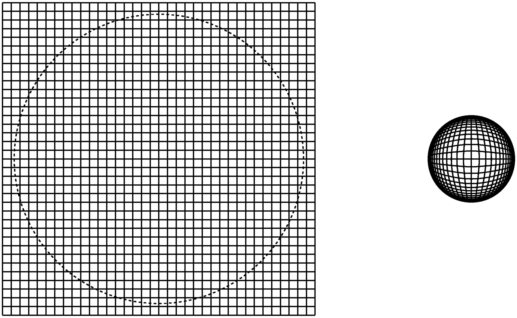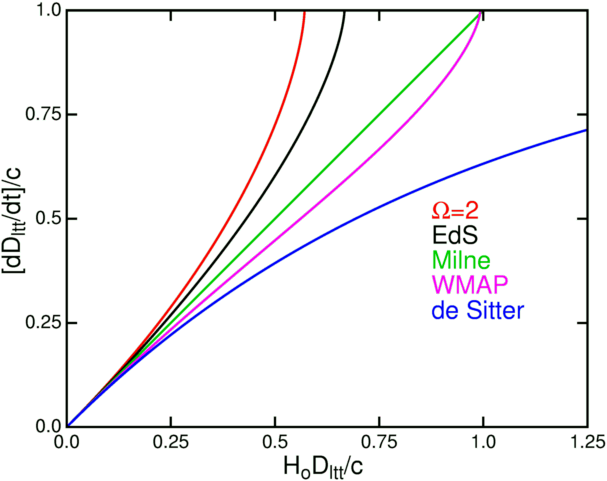# Why the Light Travel Time Distance should not be used in Press Releases

Since public information offices in the US never want to mention the redshift of an object, distances are usually given as light travel time distances. This has one simple property: the distance in light years is never greater than the age of the Universe in years, avoiding at least one appearance of speeds greater than the speed of light.

This doesn't really satisfy the simplicity requirement, because a numerate listener will ask: if a distant cluster of galaxies is 9.1 billion light years away in a universe that is 13.7 billion years old, how did the cluster get so far away in only 4.6 billion years? If must have been travelling faster than the speed of light! Apparently it takes several minutes for this question to arise, and by that time the presenters are out the door. So I get an E-mail.

So here I will list the reasons why this press office policy is really dumb:

• The redshift z is usually the only number in the whole story that is unambiguous and likely to be correct.
• Distance is defined as the spatial separation at a common time. It makes no sense to talk about the difference in spatial positions of a distant galaxy seen 9.1 billion years ago and the Milky Way now when galaxies are moving.
• If an SR-71 blackbird flies over at Mach 3 and you hear the sound 30 seconds later, then answer to the question "How far away is it?" is clearly not 30 "sound seconds" or 10 km.
• The Universe is homogeneous and isotropic, so it has no edge. Thus there cannot be a maximum distance. Distances greater than speed of light times the age of the Universe are commonplace. But a uniform grid in the Universe shown at left below is very non-uniform when plotted using the light travel time distance, as shown at right below:• The Hubble law is satisfied exactly for "distance now", or metric radial distance. In fact the Hubble law with time variable Hubble parameter is satisfied exactly at all times by the metric radial distance D(t) which is the spatial separation at the common time t, so
"velocity" = dD/dt = H(t)D(t).
This is not true for the light travel time distance. The rate of change of the light travel time distance with observation time is always
dDltt/dt = cz/(1+z)
which generally disagrees with the Hubble law as shown below:As a service to astronomers who are frustrated by press releases that give only light travel times and not redshifts, here is a version of cosmology calculator that takes the light travel time and computes the redshift. Of course you need to know the cosmological parameters the PAO used when hiding the useful information, but this calculator defaults to the WMAP 1st year Ho = 71 and OmegaM = 0.27 flat Lambda-CDM cosmology.